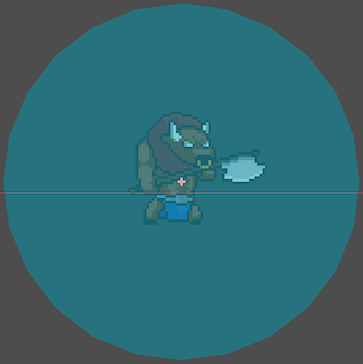# Chasing the player

## Problem

You want an enemy to chase the player.

## Solution

The first step in getting an enemy to chase the player is to determine what direction the enemy needs to move. To get the vector pointing from A to B, you subtract: B - A. Normalize the result and you have a direction vector.

This makes the solution quite straightforward. Every frame, set the enemy’s velocity to point in the direction of the player.

``````velocity = (player.position - position).normalized() * speed
``````

Godot’s `Vector2` object has a built-in helper for this:

``````velocity = position.direction_to(player.position) * speed
``````

However, this would allow the enemy to chase the player from any distance, even if it’s far away. To fix this, we can add an `Area2D` to the enemy, and only chase the player when it’s inside this “detect radius”.Here’s some example code:

``````extends KinematicBody2D

var run_speed = 25
var velocity = Vector2.ZERO
var player = null

func _physics_process(delta):
velocity = Vector2.ZERO
if player:
velocity = position.direction_to(player.position) * run_speed
velocity = move_and_slide(velocity)

player = body

player = null
``````

We’ve connected the `body_entered` and `body_exited` signals from the `Area2D` so that the enemy knows whether it’s in range or not.

This concept can be extended to other types of games as well. The key is to find the direction vector from the enemy to the player:

``````# 2D
(player.position - position).normalized()
# 3D
(player.transform.origin - transform.origin).normalized()
``````

If, for example, your game is a side-scroller or has other constraints in movement, you can use only the `x` component of the resulting vector to determine movement.

### Limitations

Note that this method results in very simplistic straight-line movement. The enemy will not move around obstacles such as walls, nor will it stop if it gets too close to the player.

Another problem is more apparent with fast-moving enemies. As the player moves, the enemies using this technique will change direction instantly. For a more natural-looking movement, you’ll want to use a steering behavior.

For more advanced behaviors, see the other recipes in this chapter.